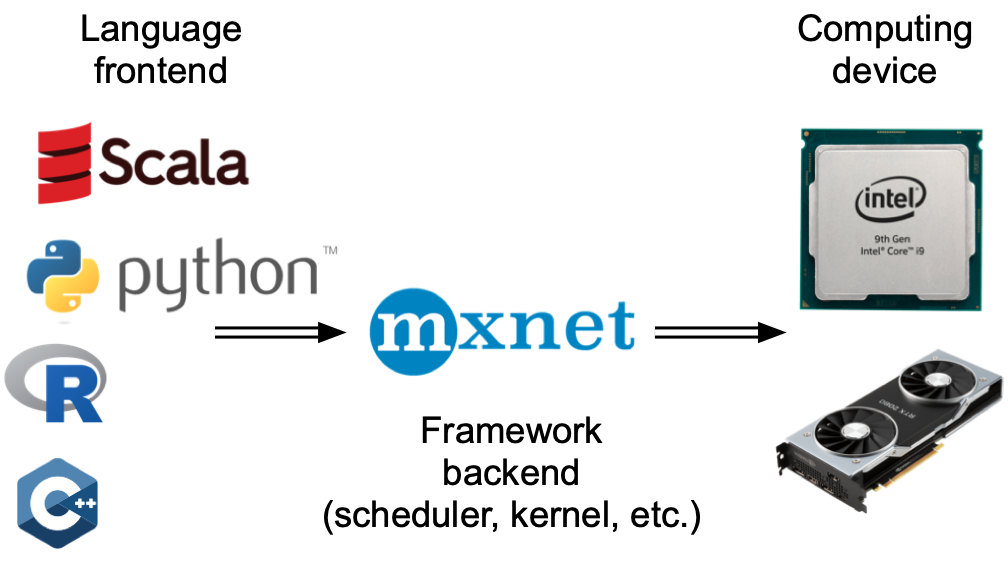# 12.2. 异步计算¶ Open the notebook in Colab Open the notebook in Colab Open the notebook in Colab Open the notebook in SageMaker Studio Lab

import os
import subprocess
import numpy
from mxnet import autograd, gluon, np, npx
from mxnet.gluon import nn
from d2l import mxnet as d2l

npx.set_np()

import os
import subprocess
import numpy
import torch
from torch import nn
from d2l import torch as d2l


## 12.2.1. 通过后端异步处理¶

with d2l.Benchmark('numpy'):
for _ in range(10):
a = numpy.random.normal(size=(1000, 1000))
b = numpy.dot(a, a)

with d2l.Benchmark('mxnet.np'):
for _ in range(10):
a = np.random.normal(size=(1000, 1000))
b = np.dot(a, a)

numpy: 1.2820 sec
mxnet.np: 0.0163 sec


with d2l.Benchmark():
for _ in range(10):
a = np.random.normal(size=(1000, 1000))
b = np.dot(a, a)
npx.waitall()

Done: 1.0323 sec


# GPU计算热身
device = d2l.try_gpu()
a = torch.randn(size=(1000, 1000), device=device)
b = torch.mm(a, a)

with d2l.Benchmark('numpy'):
for _ in range(10):
a = numpy.random.normal(size=(1000, 1000))
b = numpy.dot(a, a)

with d2l.Benchmark('torch'):
for _ in range(10):
a = torch.randn(size=(1000, 1000), device=device)
b = torch.mm(a, a)

numpy: 1.2837 sec
torch: 0.0010 sec


with d2l.Benchmark():
for _ in range(10):
a = torch.randn(size=(1000, 1000), device=device)
b = torch.mm(a, a)
torch.cuda.synchronize(device)

Done: 0.0106 secx = np.ones((1, 2))
y = np.ones((1, 2))
z = x * y + 2
z

array([[3., 3.]])

x = torch.ones((1, 2), device=device)
y = torch.ones((1, 2), device=device)
z = x * y + 2
z

tensor([[3., 3.]], device='cuda:0')## 12.2.2. 障碍器与阻塞器¶

• 最明显的是，npx.waitall()不考虑计算指令的发出时间，等待直到所有计算完成。除非绝对必要，否则在实践中使用此运算符不是个好主意，因为它可能会导致较差的性能。

• 如果只想等待一个特定的变量可用，我们可以调用z.wait_to_read()。在这种情况下，MXNet阻止程序返回Python，直到计算出变量z为止。z之后的其他计算才可能很好地继续。

with d2l.Benchmark('waitall'):
b = np.dot(a, a)
npx.waitall()

b = np.dot(a, a)

waitall: 0.0049 sec


with d2l.Benchmark('numpy conversion'):
b = np.dot(a, a)
b.asnumpy()

with d2l.Benchmark('scalar conversion'):
b = np.dot(a, a)
b.sum().item()

numpy conversion: 0.0064 sec
scalar conversion: 0.0186 sec


## 12.2.3. 改进计算¶

with d2l.Benchmark('synchronous'):
for _ in range(10000):
y = x + 1

with d2l.Benchmark('asynchronous'):
for _ in range(10000):
y = x + 1
npx.waitall()

synchronous: 1.2496 sec
asynchronous: 0.8348 sec


Python前端线程和C++后端线程之间的简化交互可以概括如下：

1. 前端命令后端将计算任务y = x + 1插入队列。

2. 然后后端从队列接收计算任务并执行。

3. 然后后端将计算结果返回到前端。 假设这三个阶段的持续时间分别为$$t_1, t_2, t_3$$。如果不使用异步编程，执行10000次计算所需的总时间约为$$10000 (t_1+ t_2 + t_3)$$。如果使用异步编程，因为前端不必等待后端为每个循环返回计算结果，执行$$10000$$次计算所花费的总时间可以减少到$$t_1 + 10000 t_2 + t_3$$（假设$$10000 t_2 > 9999t_1$$）。

## 12.2.4. 小结¶

• 深度学习框架可以将Python前端的控制与后端的执行解耦，使得命令可以快速地异步插入后端、并行执行。

• 异步产生了一个相当灵活的前端，但请注意：过度填充任务队列可能会导致内存消耗过多。建议对每个小批量进行同步，以保持前端和后端大致同步。

• 芯片供应商提供了复杂的性能分析工具，以获得对深度学习效率更精确的洞察。

• 将MXNet管理的内存转换到Python将迫使后端等待特定变量就绪，printasnumpyitem等函数也具有这个效果。请注意，错误地使用同步会破坏程序性能。

## 12.2.5. 练习¶

1. 我们上面提到，使用异步计算可以将执行$$10000$$次计算所需的总时间减少到$$t_1 + 10000 t_2 + t_3$$。为什么我们要假设这里是$$10000 t_2 > 9999 t_1$$

2. 测量waitallwait_to_read之间的差值。提示：执行多条指令并同步以获得中间结果。

Discussions

1. 在CPU上，对本节中相同的矩阵乘法操作进行基准测试。你仍然可以通过后端观察异步吗？

Discussions